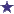# Pharmacy IV Math Question: Weight/Volume Dextrose in Water to Add to 500cc D10W

by Don
(Seattle)IV Dextrose in Water-Weight/Volume Pharmacy Math Question

A pharmacist needs to compound an IV which is 8% w/v dextrose in water. How many cc of pure sterile water should she add to 500cc of D10W to make the IV?

I would appreciate the help!

### Comments for Pharmacy IV Math Question: Weight/Volume Dextrose in Water to Add to 500cc D10W

Average RatingRatingDilution / Compounding Pharmacy Math Question... by: David To start with, D10W is 10% Dextrose in Sterile Water. We have 500cc or MLs of this hydration. What we are trying to make or compound is 8% Dextrose in Sterile Water. This is a dilution math problem. Keep in mind, 10% Dextrose in 500ML of Sterile water is percent weight in volume (w/v). This means that weight (in this question, 10 grams (w/v is figured per 100ML of liquid) in volume = 50gms/500ML = 10%. What we want to get to is 8% weight in volume. How much sterile water do we need to add (volume) to get to this 8%? 8% is what decimal? 0.125 10% (50gm in 500ML) 50gm 50gm ---- = ---------------- = 8% = 0.125 500ML+R H = (500ML + R) Where "R" is the sterile water volume to add and "H" is the final volume. We want to dilute this solution 2%, from 10% to 8%. So we plug these values into the equation: 50gm ---------------------------------- 500ML + 125ML = 625ML How does this convert into a percentage? 625ML X 0.08 (8%) = 50gm 8% = 625ML times 0.125 = 50gms You (or your pharmacist) will need to add 125ML of Sterile water to the 500ML of D10W, making a total volume of 625ML. 8% of 625ML = 50GM.